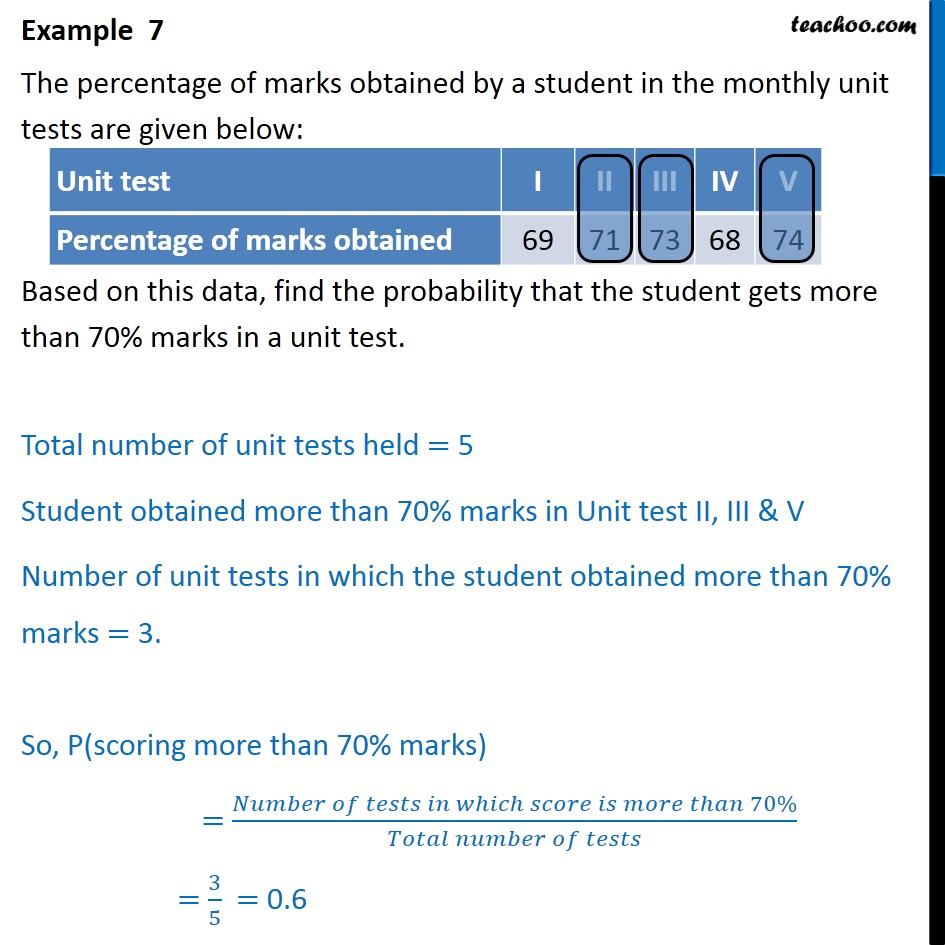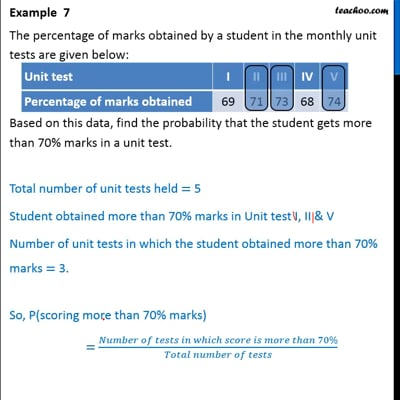Examples

Chapter 15 Class 9 Probability (Deleted)
Serial order wiseThis video is only available for Teachoo black users

Solve all your doubts with Teachoo Black (new monthly pack available now!)

### Transcript

Example 7 The percentage of marks obtained by a student in the monthly unit tests are given below: Based on this data, find the probability that the student gets more than 70% marks in a unit test. Total number of unit tests held = 5 Student obtained more than 70% marks in Unit test II, III & V Number of unit tests in which the student obtained more than 70% marks = 3. So, P(scoring more than 70% marks) = 3 5 = 0.6